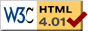### Journée-séminaire de combinatoire

#### (équipe CALIN du LIPN, université Paris-Nord, Villetaneuse)

Le 21 octobre 2019 à 14h50 en Amphi Darwin, Jacopo Borga nous parlera de : Phase transition for almost square permutations

Résumé : A record in a permutation is an entry which is either bigger or smaller than the entries either before or after it (there are four types of records). Entries which are not records are called internal points. We explore scaling limits (called permuton limits) of uniform permutations in the classes Sq(n,k) of almost square permutations of size n+k with exactly k internal points. We first investigate the case when k=0, this is the class of square permutations, i.e. permutations where every point is a record. The starting point for our result is a sampling procedure for asymptotically uniform square permutations. Building on that, we characterize the global behavior by showing that square permutations have a permuton limit which can be described by a random rectangle. We also explore the fluctuations of this random rectangle, which we can describe through coupled Brownian motions. We then characterize the permuton limit of almost square permutations with k internal points, both when k is fixed and when k tends to infinity along a negligible sequence with respect to the size of the permutation. Here the limit is again a random rectangle but of a different nature: we will show during the seminar that a phase transition on the shape of the limiting rectangles arises for different values of k. Joint work with E.Slivken and E. Duchi.

 Dernière modification : Monday 24 January 2022Contact pour cette page : Cyril.Banderier at lipn.univ-paris13.fr